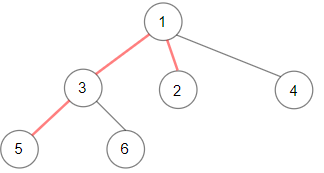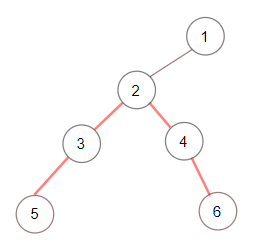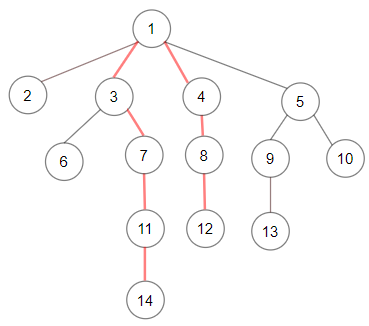Formatted question description: https://leetcode.ca/all/1522.html

# 1522. Diameter of N-Ary Tree

Medium

## Description

Given a root of an N-ary tree, you need to compute the length of the diameter of the tree.

The diameter of an N-ary tree is the length of the longest path between any two nodes in the tree. This path may or may not pass through the root.

(Nary-Tree input serialization is represented in their level order traversal, each group of children is separated by the null value.)

Example 1:Input: root = [1,null,3,2,4,null,5,6]

Output: 3

Explanation: Diameter is shown in red color.

Example 2:Input: root = [1,null,2,null,3,4,null,5,null,6]

Output: 4

Example 3:*Input:8 root = [1,null,2,3,4,5,null,null,6,7,null,8,null,9,10,null,null,11,null,12,null,13,null,null,14]

Output: 7

Constraints:

• The depth of the n-ary tree is less than or equal to 1000.
• The total number of nodes is between [0, 10^4].

## Solution

The diameter of an N-ary tree is the sum of the to maximum depths in its subtrees. Use recursion to obtain the depths and calculate the diameter.

/*
// Definition for a Node.
class Node {
public int val;
public List<Node> children;

public Node() {
children = new ArrayList<Node>();
}

public Node(int _val) {
val = _val;
children = new ArrayList<Node>();
}

public Node(int _val,ArrayList<Node> _children) {
val = _val;
children = _children;
}
};
*/

class Solution {
int path;

public int diameter(Node root) {
path = 1;
depthFirstSearch(root);
return path - 1;
}

public int depthFirstSearch(Node node) {
if (node == null)
return 0;
int maxDepth1 = 0, maxDepth2 = 0;
List<Node> children = node.children;
for (Node child : children) {
int curDepth = depthFirstSearch(child);
if (curDepth > maxDepth1) {
maxDepth2 = maxDepth1;
maxDepth1 = curDepth;
} else if (curDepth > maxDepth2)
maxDepth2 = curDepth;
path = Math.max(path, maxDepth1 + maxDepth2 + 1);
}
return maxDepth1 + 1;
}

public int depthFirstSearch(TreeNode node) {
if (node == null)
return 0;
int leftDepth = depthFirstSearch(node.left);
int rightDepth = depthFirstSearch(node.right);
path = Math.max(path, leftDepth + rightDepth + 1);
return Math.max(leftDepth, rightDepth) + 1;
}
}